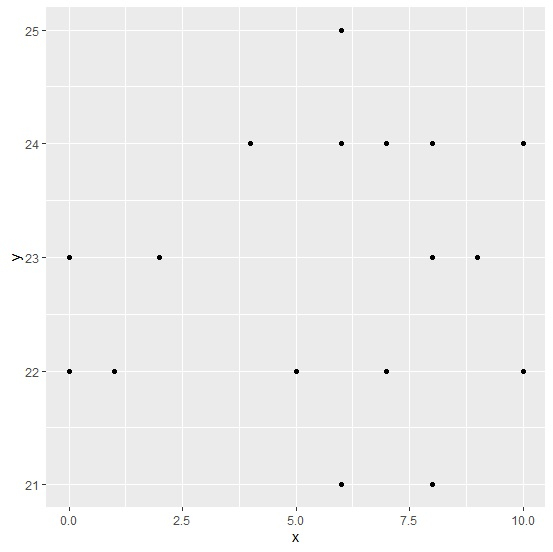# How to deal with warning message ‚ÄúRemoved X rows containing missing values‚Äù for a column of an R data frame while creating a plot?

If we have missing values/NA in our data frame and create a plot using ggplot2 without excluding those missing values then we get the warning “Removed X rows containing missing values”, here X will be the number of rows for the column that contain NA values. But the plot will be correct because it will be calculated by excluding the NA’s. To avoid this error, we just need to pass the subset of the data frame column that do not contains NA values as shown in the below example.

Consider the below data frame with y column having few NA values −

## Example

Live Demo

set.seed(112)
x<-sample(0:10,25,replace=TRUE)
y<-sample(c(21:25,NA),25,replace=TRUE) df<-data.frame(x,y)
df

## Output

   x  y
1  4  21
2  10  NA
3  10  23
4  10 22
5  2  NA
6  1 NA
7  0 25
8  8 NA
9  1 22
10 4 23
11 2 21
12 3 23
13 9 25
14 6 25
15 7 21
16 10 24
17 6 NA
18 6 NA
19 8 NA
20 4 24
21 1 23
22 7 21
23 1 21
24 0 22
25 4 NA

Loading ggplot2 package and creating point chart for x and y columns of df −

library(ggplot2) ggplot(df,aes(x,y))+geom_point()

Warning message −

Removed 5 rows containing missing values (geom_point) −

Here, we are getting the warning message for missing values.

## Plot OutputCreating the point chart for x and y by excluding the NA values −

## Example

ggplot(data=subset(df,!is.na(y)),aes(x,y))+geom_point()

Output of the plot would be same as shown above but the warning message will not be there −Updated on: 16-Oct-2020

556 Views